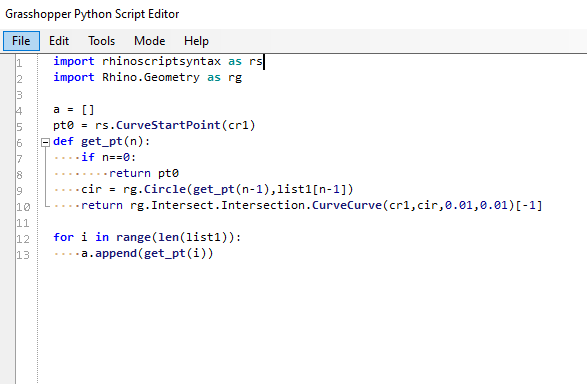# Divide distance的功能

grasshopper中divide distance只能输入固定的一个数。如果想要根据自己的列表分割比如[1,1.2,1.5,1.1]，相邻点的间隔是列表中的数据，怎么达到呢？

2 个赞
1 个赞import rhinoscriptsyntax as rs
import Rhino.Geometry as rg

a=[]
pt0 = rs.CurveStartPoint(cr1)

def get_pt(n):
if n==0:
return pt0
return rs.IntersectBreps(cr1, cir)[-1]

for i in range(x):
a.append(get_pt(i))

``````import rhinoscriptsyntax as rs
import Rhino.Geometry as rg

#定义一个列表装生成的点
a=[]

#运行的次数
count = 0
curve_start = rs.CurveStartPoint(crv)
domain = rs.CurveDomain(crv)
a.append(curve_start)

while(True):
#判断取值索引是否超出列表
if(count>=len(dis)):count %=len(dis)
#创建圆
#求交点
intersection_list = rs.CurveCurveIntersection(crv,circle)
#跳出循环的条件
if(intersection_list==None):break
#求曲线上的相交点和相交点参数t
curve_start,t = intersection_list,intersection_list
a.append(curve_start)

#创建创建子曲线，进行循环